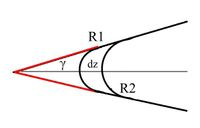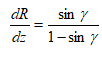Inspico High Resolution Analysis
Dedicated Instruments:  Field Ion Microscopy & Atom Probe Tomography

# TaperGeometry

The tip radius is determined omitting the varying evaporation field strength. Instead of determining the radius by the applied voltage, the radius is determined as shown in the following Figurewhere γ represents the shaft angle of the tip. Thus, the actual tip radius is determined by number of evaporated atoms or in other words by the achieved depth of analysis. Only the initial radius of curvature and the shaft angle have to be known. These parameters can be determined by TEM analysis. On the other hand, the evaporation field strength can be calculated using 2‑1. From the change of evaporation field strength, the correctness of the used parameters can be verified

Pseudo - Code

Detector Size A
Detector Efficiency p
Image Compression Factor κ
Field Compression Factor β
Taper Angle α
Use Depth Scaling Aperture ϴ

/* Taper Geometry

apert = ϴ
if(ϴ is not checked)
ang = sqrt(A/π)
apert = ang/(L * κ)
if(curv is not checked)
curv = 0
for(every event)
phi = atan2(Yd,Xd)
theta = sqrt(Xd *Xd + Yd * Yd)/L)/κ

Xi = Ri * sin(theta) * cos(phi)
Yi = Ri * sin(theta) * sin(phi)
Zi = T + Ri * (1 - cos(theta))

Ui = Ubi + c * Upi
E = Ui/(β * Ri)
if(theta < ϴ AND d =! 0)
w = sin(α)/(1-sin(α))
a = sin(apert) * sin(apert) - w * (1 -cos(apert)) * (1 -cos(apert))
dz = 1/(π * R * R * p * d * a)
T = T + dz
R = R + dz * w
α = α + dz * curv
*/